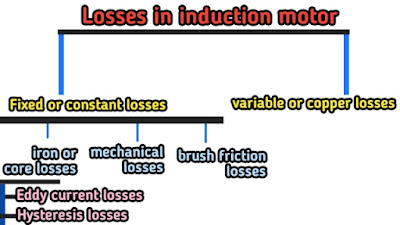# Losses in induction motor, Efficiency, Crawling And Cogging

Hello guys welcome to my blog. Hope you are all good and well.

• Different types of losses of induction motor.
• Efficiency of induction motor.
• Crawling and cogging.
•

Now come on to learn about,

# Losses and efficiency of induction motor: -

## Losses in induction motor: -

Hence we know that there are various losses occurring in the induction motor, but the most common type of losses in induction motors are: -
1. Fixed losses or constant losses
2. Variable losses or copper lossesFig. Losses in induction motor

Now let's come to discuss about these losses in the brief.

## 1. Fixed or constant losses: -

#### There are mainly three types of fixed losses in induction motor are: -

A). Iron losses or core losses
B). Windage losses
C). Brushes friction losses

*The most common type of fixed or constant loss are iron losses or core losses.

### A). Iron loss or core loss: -

#### Eddy current losses: -

Eddy current losses are the losses which occurs due to the eddy current (rotating current) flow through the core.

When the Eddy current flow in the core the heat is produced within the core in the form of heat, this heat is known as Eddy current losses.

#### Hysteresis losses: -

The hysteresis loss occurs due to the magnetizing and demagnetizing of the core and due to this magnetizing and demagnetizing of core, heat is produced (in the core) in the form of losses and known as hysteresis loss.

Since both these losses depend upon the frequency which is almost constant in all the time, therefore these losses are called fixed or constant losses.

Note: -
The frequency of the stator is always supply frequency and the frequency of the rotor is slip times of the supply frequency i.e. S×f which is always less than the stator frequency.

Hence the rotor core losses are very small as compared to stator core losses and is usually neglected in running conditions.

So,

### How to reduce these losses in induction motor??

The Eddy current losses and hysteresis losses can be minimized by using silicon steel laminations to make the core of induction motor.

High grade silicon steel is used to reduce the hysteresis losses where are the laminations are used to reduce Eddy current losses.

So a question arises here that why laminations are used to reduce the Eddy current losses?

As we know that the resistance is inversely proportional to the area of the core, so if we use laminations the area of core reduce and therefore the resistance of the core will increase and further due to increase in resistance the flow of Eddy current is reduced. Therefore laminations are used to reduce the Eddy current losses.

### B). Windage losses :-

As the name suggests, these are the losses which occur due to the wind produce during the working of the induction motor.

When motor run at a speed, then the wind will produce, which oppose the rotor speed direction and decrease its speed. But here it should be noted that windage losses are negligible.

### C). Brush friction losses: -

These losses are occur in the motor due to the friction of the brushes and bearing. And can be minimized by proper lubrication.

## 2. Variable losses or copper losses :-

These are the losses occurs due to the current flowing in the stator and rotor windings.
As the load changes the current flowing in the rotor and stator windings also changes and hence these losses will also change therefore these losses are called variable losses.
The copper losses are obtained by performing block rotor tests of induction motor.

(We will provide link soon for test of three phase induction motor).

## Efficiency of induction motor :-

As we know that the efficiency is the ratio of output power to the input power.

Efficiency = output power / input power

So the efficiency of three phase induction motors is the ratio of power developed at the shaft to the electrical power input given to the motor.

## Faults occur in an induction motor: -

There are various faults occuring in an induction motor. Some faults are-
Earth fault
• Over voltage
• Under voltage
• Short circuit
• Stalling
• Ambient high temperature
• Improper lubrications
• Wrong alignment
• Excessive vibrations

Now let’s come to learn about two basic definitions used in induction motors: -

1. Crawling
2. Cogging

## Crawling and Cogging: -

### 1. Crawling of induction motor: -

The squirrel cage induction motor tends to rotate at a very low speed as compared to its synchronous speed. This phenomenon is known as crawling of induction motor.

### 2. Cogging of induction motor: -

Sometimes the induction motor refuse to start or to rotate at all times. The reason behind this may be low supply voltage and cogging.

But the main reason behind this, is cogging in which slots of the starter get locked up with the slots of the rotor.

As we know that, there are several slots in the stator and rotor of induction motor when the slots of the rotor are equal with slot in the stator they align themselves in such a way that they both face to each and refuse to start. This phenomenon is called cogging.

This is also known as magnetic teeth locking of induction motor.

So a question arises here that,

### How to reduce cogging?

There are two methods to reduce cogging: -

1. The number of slots in the rotor should not be equal to the number of slots in the stator.

2. By skewing of the rotor slots.